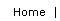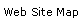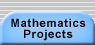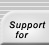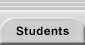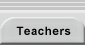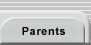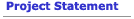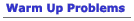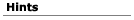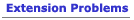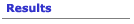## Hints for the Simplex Lock Problem

Most solutions to the Simplex Lock Problem break down into two categories:

• effective enumerations, and
• combinatorial arguments.

#### 1 Enumeration Strategies

When working on a problem that involves counting, especially counting complicated things like these combinations, you have to pay attention to two fundamental rules of counting:

1. Count everything once.
2. Count nothing more than once.

These rules may seem obvious, but they’re not always easy to implement. In this case, if you’re actually going to count every combination, you need a way to list them systematcially, to be sure you don’t miss anything and that you don’t list things twice.

Here are some ideas for organization. These are just ideas. Pick one or two of them that appeals to you and pursue them. You might want to combine two or more strategies. For example, you can start with locks that have fewer than five buttons, and use one of the other strategies suggested to count combinations on those locks. Or these strategies might give you an idea for a totatlly different approach. That’s great!

#### 1.1 Buttons

Classify the combinations by how many buttons they use.
• There are five combinations that use only one button.
• Combinations that use only two buttons come in two types: press one button then press another; or press two buttons together. How many of each kind are there?

#### 1.2 Presses

Break down the possible combinations into how many “presses” they have. One press means pressing down one or more buttons simultaneously.
• So the combination 2|3 4 5|1 is three presses. The combination 1|2|3 is also three presses.
• If a combination uses five presses, it must use all five of the buttons in some order. 1|2|3|4|5, 2|1|3|4|5, etc.

#### 1.3 Shape

Look at the shape of the combinations. How many look like * * |*, or *| * *|*? Working on the warm-up project might help with this strategy.

#### 1.4 Fewer Buttons

Work on locks with fewer buttons.
• It’s easy to count the combinations for a 1-button lock; there are two combinations: Press the button, or don’t press it (the door is unlocked).
• Then count the combinations for a 2-button lock. Look for patterns in how the number of combinations grows.

#### 1.5 Computer Program

Write a computer program to generate the actual combinations. Then all you have to do is count them. (You could even have the program count them as it generates them!)

#### 1.6 Extension

Make a list of all the combinations on a four-button lock and come up with a systematic way to extend these to the combinations on a five button lock. For example, here’s a combination on a 4-button lock (it doesn’t use all 4 buttons, but it is still a valid combination for that lock):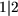If you add in button 5, you get all of these combinations: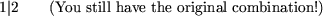Can you find a way to extend all the 4-button combinations to combinations on the 5-button lock without duplication and without leaving anything out?

#### 1.7 Buttons 2

The combinations come in two types: those that use all five buttons and those that use fewer. Count these separately.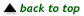#### 2 Combinatorial Strategies

If you know a bit about combinatorics, you can use what you know to shorten the work of counting the combinations on the lock.

#### 2.1 Shape

First, a standard piece of notation: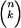(read this as “n choose k”) is the number of ways to pick k objects from a set of n objects.

In the case of the lock,(“5 choose 3”) would be the number of ways to pick 3 buttons from the set of 5. If you were counting combinations where you push 3 buttons at once and that’s all, there would be exactly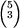combinations.

To calculate, you use the equation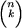=. So for, you get=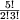= 10. On a 5-button lock, there are 10 combinations formed by pushing 3 buttons at once.

Here are the shapes of pushes that use 3 buttons: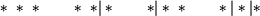The number of combinations of the first shape is exactly the number of ways to choose 3 buttons out of 5, or. To make combinations of the second shape, you first pick 2 buttons out of 5, then pick one button from the remaining 3. So the total number is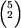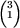.

If you can find the shape of each possible combination, you can use these combinatorial formulas to find the number of combinations of each shape.

#### 2.2 Decomposition

Let c(n) be the number of combinations on an n-button lock. You can break c(n) down into the number of combinations that use 1 press, 2 presses, and so on up to n presses. So define another function p(k, n) to be the number of combinations on an n-button lock that use exactly k presses. So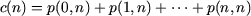Suppose you have p(1, n 1). That is, you have all the combinations on n - 1 buttons that use k - 1 presses. To make it into combinations using n buttons and k presses, you can insert that last button into any of the “holes.” There are k ways to do this.

An example: Here are some of the combinations using 3 buttons, 2 presses.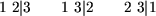We’ll change just the first one into combinations using 4 buttons and 3 presses. Notice there are 3 ways to do this. (You could do the same with each of the other combinations.)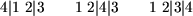Now, suppose you have p(k, n 1). That is, you have all the combinations on 1 buttons that use presses. To make it into combinations using buttons and k presses, you can add the last button into any of the existing k presses, or you can leave it alone (you don’t have to use all the buttons). There are a total of + 1 new combinations.

An example: Here are some of the combinations using 3 buttons and 3 presses:We’ll change just the first one into combinations using 4 buttons and 3 presses. Notice there are 4 ways to do this. (You could do the same with each of the other combinations.)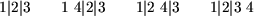With a little more reasoning, you can show that this equation is true: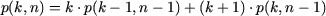(You need to show that the two strategies above get you all of the combinations on n buttons that use k presses--that you don’t leave any out.)

With this relationship, you could devise a recursive function for p(n, k) and use it to count p(0, 5), p(1, 5), and so on.

#### 2.3 Extension

If you have a combination that uses k pushes, then you can extend it in k + k + 1 ways to a combiantion using one more button. You can add the new button into an existing push (in k ways) or between existing pushes (in + 1 ways).

An example: Here’s a combination that uses 2 pushes: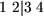Add another button in an existing push: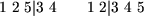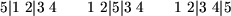Translations of mathematical formulas for web display were created by tex4ht. © Copyright 2003 Education Development Center, Inc. (EDC)